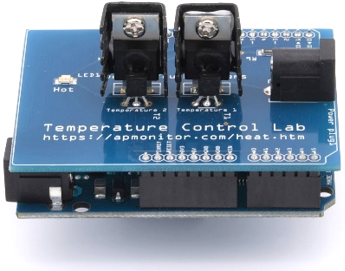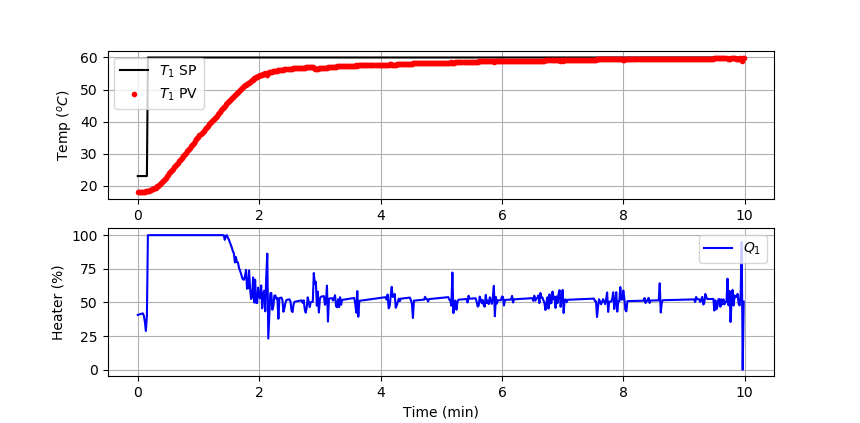## TCLab PID ControlObjective: Implement a discrete PID controller and test the performance with a setpoint change from 23oC to 60oC. Use the IMC aggressive tuning correlation.

A PID controller is an equation that adjusts the controller output, Q(t). It is a calculation of the difference between the setpoint T_{SP} and process variable T_{PV}. The adjustable parameters are the controller gain, K_c, and controller reset time or integral time constant, \tau_I. A large gain or small integral time constant produces a controller that reacts aggresively to a difference between the measured PV and target SP.

$$e(t) = T_{SP}-T_{PV}$$

$$Q(t) = Q_{bias} + K_c \, e(t) + \frac{K_c}{\tau_I}\int_0^t e(t) dt - K_c \tau_D \frac{d\left(T_{PV}\right)}{dt}$$

The Q_{bias} term is a constant that is typically set to the value of Q(t) when the controller is first switched from manual to automatic mode. The deviation variable for the heater is the change in value Q'(t) = Q(t) - Q_{bias}. For the TCLab, Q_{bias}=0 because the TCLab starts with the heater off. The Q_{bias} gives bumpless transfer if the error is zero when the controller is turned on. The continuous integral is approximated in a discrete form as a summation of the error multiplied by the sample time.

$$\frac{K_c}{\tau_I}\int_0^t e(t) dt \approx \frac{K_c}{\tau_I} \sum_{i=1}^{n_t} e_i(t)\Delta t$$

Likewise, the derivative term is a finite difference approximation. A common method uses the difference between the current and prior values of the PV to get the current slope.

$$\frac{d\left(T_{PV}\right)}{dt} \approx \frac{T_{PV,n_t}-T_{PV,n_t-1}}{\Delta t}$$

One potential problem with this form is that measurement noise is amplified and can cause controller output fluctuations. One way to reduce this is to use a first order filter or linear regression from the prior n time points. The PID equation in discrete form is the following:

$$Q(t) = Q_{bias} + K_c \,e(t) + \frac{K_c}{\tau_I} \sum_{i=1}^{n_t} e_i(t)\Delta t - K_c \tau_D \frac{T_{PV,n_t}-T_{PV,n_t-1}}{\Delta t}$$

Python PID Function

This equation can be implemented in Python as a function with inputs:

• sp = setpoint
• pv = current temperature
• pv_last = prior temperature
• ierr = integral error
• dt = time increment between measurements

and outputs:

• op = output of the PID controller
• P = proportional contribution
• I = integral contribution
• D = derivative contribution

The output Integral contribution (I) must be fed back in as an input to the function each successive call. The tuning parameters Kc, tauI, and tauD are defined as global variables or they could also be switched to function inputs.

def pid(sp,pv,pv_last,ierr,dt):
# Parameters in terms of PID coefficients
KP = Kc
KI = Kc/tauI
KD = Kc*tauD
# ubias for controller (initial heater)
op0 = 0
# upper and lower bounds on heater level
ophi = 100
oplo = 0
# calculate the error
error = sp-pv
# calculate the integral error
ierr = ierr + KI * error * dt
# calculate the measurement derivative
dpv = (pv - pv_last) / dt
# calculate the PID output
P = KP * error
I = ierr
D = -KD * dpv
op = op0 + P + I + D
# implement anti-reset windup
if op < oplo or op > ophi:
I = I - KI * error * dt
# clip output
op = max(oplo,min(ophi,op))
# return the controller output and PID terms
return [op,P,I,D]

IMC Aggressive Tuning

Use an IMC aggressive tuning correlation with FOPDT parameters (K_c, \tau_p, \theta_p) determined from the TCLab graphical fitting or TCLab regression exercises.

$$\mathrm{Aggressive\,Tuning:} \quad \tau_c = \max \left( 0.1 \tau_p, 0.8 \theta_p \right)$$

$$K_c = \frac{1}{K_p}\frac{\tau_p+0.5\theta_p}{\left( \tau_c + 0.5\theta_p \right)} \quad \quad \tau_I = \tau_p + 0.5 \theta_p \quad \quad \tau_D = \frac{\tau_p\theta_p}{2\tau_p + \theta_p}$$

Fill in the values of K_c, \tau_I, \tau_D, and the PID equation in the code below. Use anti-reset windup to prevent the integral from accumulating when the heater is saturated at 0% or 100%. Show a plot of the SP (temperature setpoint), PV (measured temperature), and OP (heater value) and report the integral absolute error between the setpoint and measured temperature.

PID Control Starting Script

import numpy as np
import matplotlib.pyplot as plt
import tclab
import time

# -----------------------------
# Input Kc,tauI,tauD
# -----------------------------
Kc =
tauI =
tauD =

#------------------------
# PID Controller Function
#------------------------
# sp = setpoint
# pv = current temperature
# pv_last = prior temperature
# ierr = integral error
# dt = time increment between measurements
# outputs ---------------
# op = output of the PID controller
# P = proportional contribution
# I = integral contribution
# D = derivative contribution
def pid(sp,pv,pv_last,ierr,dt):
# Parameters in terms of PID coefficients
KP = Kc
KI = Kc/tauI
KD = Kc*tauD
# ubias for controller (initial heater)
op0 = 0
# upper and lower bounds on heater level
ophi = 100
oplo = 0
# calculate the error
error = sp-pv
# calculate the integral error
ierr = ierr + KI * error * dt
# calculate the measurement derivative
dpv = (pv - pv_last) / dt
# calculate the PID output
P = KP * error
I = ierr
D = -KD * dpv
op = op0 + P + I + D
# implement anti-reset windup
if op < oplo or op > ophi:
I = I - KI * error * dt
# clip output
op = max(oplo,min(ophi,op))
# return the controller output and PID terms
return [op,P,I,D]

n = 600  # Number of second time points (10 min)
tm = np.linspace(0,n-1,n) # Time values
lab = tclab.TCLab()
T1 = np.zeros(n)
Q1 = np.zeros(n)
# step setpoint from 23.0 to 60.0 degC
SP1 = np.ones(n)*23.0
SP1[10:] = 60.0
Q1_bias = 0.0
for i in range(n):
# record measurement
T1[i] = lab.T1

# --------------------------------------------------
# call PID controller function to change Q1[i]
# --------------------------------------------------
Q1[i] =

lab.Q1(Q1[i])
if i%20==0:
print(' Heater,   Temp,  Setpoint')
print(f'{Q1[i]:7.2f},{T1[i]:7.2f},{SP1[i]:7.2f}')
# wait for 1 sec
time.sleep(1)
lab.close()
# Save data file
data = np.vstack((tm,Q1,T1,SP1)).T
np.savetxt('PID_control.csv',data,delimiter=',',\

# Create Figure
plt.figure(figsize=(10,7))
ax = plt.subplot(2,1,1)
ax.grid()
plt.plot(tm/60.0,SP1,'k-',label=r'$T_1$ SP')
plt.plot(tm/60.0,T1,'r.',label=r'$T_1$ PV')
plt.ylabel(r'Temp ($^oC$)')
plt.legend(loc=2)
ax = plt.subplot(2,1,2)
ax.grid()
plt.plot(tm/60.0,Q1,'b-',label=r'$Q_1$')
plt.ylabel(r'Heater (%)')
plt.xlabel('Time (min)')
plt.legend(loc=1)
plt.savefig('PID_Control.png')
plt.show()

See additional information on PID controllers.

Solutionimport numpy as np
import matplotlib.pyplot as plt
import tclab
import time

# process model
Kp = 0.9
taup = 175.0
thetap = 15.0

# -----------------------------
# Calculate Kc,tauI,tauD (IMC Aggressive)
# -----------------------------
tauc = max(0.1*taup,0.8*thetap)
Kc = (1/Kp)*(taup+0.5*thetap)/(tauc+0.5*thetap)
tauI = taup + 0.5*thetap
tauD = taup*thetap / (2*taup+thetap)

print('Kc: ' + str(Kc))
print('tauI: ' + str(tauI))
print('tauD: ' + str(tauD))

#------------------------
# PID Controller Function
#------------------------
# sp = setpoint
# pv = current temperature
# pv_last = prior temperature
# ierr = integral error
# dt = time increment between measurements
# outputs ---------------
# op = output of the PID controller
# P = proportional contribution
# I = integral contribution
# D = derivative contribution
def pid(sp,pv,pv_last,ierr,dt):
# Parameters in terms of PID coefficients
KP = Kc
KI = Kc/tauI
KD = Kc*tauD
# ubias for controller (initial heater)
op0 = 0
# upper and lower bounds on heater level
ophi = 100
oplo = 0
# calculate the error
error = sp-pv
# calculate the integral error
ierr = ierr + KI * error * dt
# calculate the measurement derivative
dpv = (pv - pv_last) / dt
# calculate the PID output
P = KP * error
I = ierr
D = -KD * dpv
op = op0 + P + I + D
# implement anti-reset windup
if op < oplo or op > ophi:
I = I - KI * error * dt
# clip output
op = max(oplo,min(ophi,op))
# return the controller output and PID terms
return [op,P,I,D]

n = 600  # Number of second time points (10 min)
tm = np.linspace(0,n-1,n) # Time values
lab = tclab.TCLab()
T1 = np.zeros(n)
Q1 = np.zeros(n)
# step setpoint from 23.0 to 60.0 degC
SP1 = np.ones(n)*23.0
SP1[10:] = 60.0
Q1_bias = 0.0
ierr = 0.0
for i in range(n):
# record measurement
T1[i] = lab.T1

# --------------------------------------------------
# call PID controller function to change Q1[i]
# --------------------------------------------------
[Q1[i],P,ierr,D] = pid(SP1[i],T1[i],T1[max(0,i-1)],ierr,1.0)

lab.Q1(Q1[i])
if i%20==0:
print(' Heater,   Temp,  Setpoint')
print(f'{Q1[i]:7.2f},{T1[i]:7.2f},{SP1[i]:7.2f}')
# wait for 1 sec
time.sleep(1)
lab.close()
# Save data file
data = np.vstack((tm,Q1,T1,SP1)).T
np.savetxt('PID_control.csv',data,delimiter=',',\

# Create Figure
plt.figure(figsize=(10,7))
ax = plt.subplot(2,1,1)
ax.grid()
plt.plot(tm/60.0,SP1,'k-',label=r'$T_1$ SP')
plt.plot(tm/60.0,T1,'r.',label=r'$T_1$ PV')
plt.ylabel(r'Temp ($^oC$)')
plt.legend(loc=2)
ax = plt.subplot(2,1,2)
ax.grid()
plt.plot(tm/60.0,Q1,'b-',label=r'$Q_1$')
plt.ylabel(r'Heater (%)')
plt.xlabel('Time (min)')
plt.legend(loc=1)
plt.savefig('PID_Control.png')
plt.show()